We’ve covered more vlookup problems than any other site on the internet

vlookup #N/A  error? There are FIVE different #N/A errors and two different #REF errors explained below, as well as others such as the “Invalid reference” error. If you experience a different #N/A or #REF error to the ones listed below, then tell us. We’ve discovered new errors as a result of engaging with site visitors. But please check the solutions listed below first!

This page assumes you already know how to do a vlookup – if you don’t know how to, click here for the link to the 2007/2010/2013/2016 tutorial or here for the 2003 tutorial. The file with the data for the tutorials is here – once you’ve done a vlookup in column D as explained in the tutorials, you can replicate the problems below, if you wish. You can also just review the list below to find the problem you are experiencing.

#### 1) vlookup number stored as text (#N/A error)

This is a formatting error that is very easy to fix!

If you get an “N/A#” error and there is an exclamation mark next to the unique value used for your vlookup (see cell B2 in the screenshot below) then you simply have to click on the exclamation mark box the click on “Convert to Number.”

This will change the format of the cell from “Text” to “Number” and the vlookup should work. If it still doesn’t work, read on for other N/A errors covered on this page.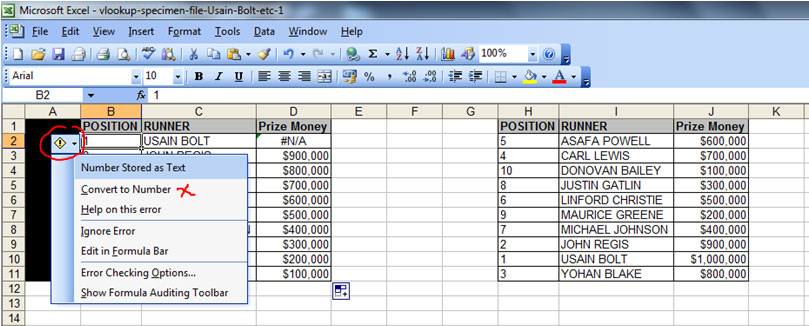#### 2) vlookup trailing spaces error AKA “the invisible dash!” (another #N/A error)

Some company reporting systems automatically generate reports where each unique value in the report has a trailing space at the end, which causes the vlookup to fail. This is the most difficult “N/A#” error to spot if you’ve never come across it before, as everything “appears” to be normal to the naked eye!

The unique values in the table below are in column B (the positions of the runners). I’ve highlighted cell B2, which has the runner in position 1 – in the toolbar, where the number “1” is shown ABOVE column C, there is a trailing space at the end (see red arrow) which caused the vlookup to fail and create an “N/A#” error in column D.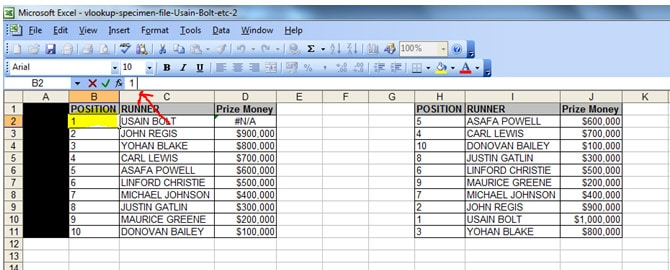There are a number of solutions for this:

2a) the “Data – ‘Text to Columns’” solution.

Go to “Data” in the tool bar, then click on the “Data” tab (it’s in between the “Formulas” and “Review” tabs).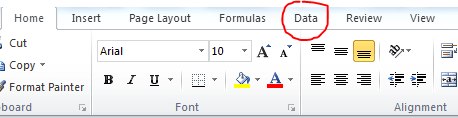Now – and this is an important step – highlight the column where your lookup values are.  In the main example on this site, the lookup values  are in column B.

Then click on the “Text to Columns” icon in the tool bar.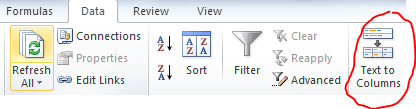Click “Next” in the window that pops up (make sure you leave “Delimited” as the file type).

Click on the “Space” checkbox in the next window (see screenshot below). The “Treat consecutive delimiters as one” checkbox will automatically get ticket when you click on the “Space” checkbox. That is fine. Just click “Next” in the screenshot below. Then click on “Finish” and that’s it!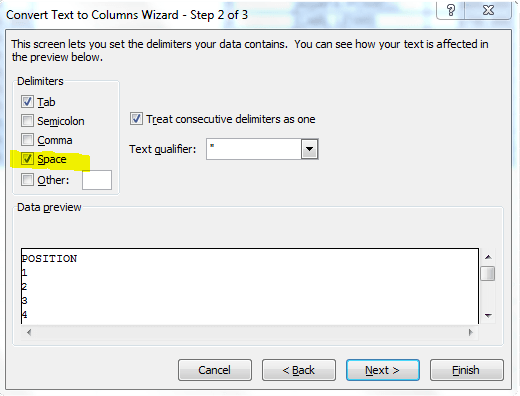2b) take out the trailing space by clicking in the cell and pressing “backspace” at the end of the cell, to remove the blank space. However, this is time-consuming.

2c) click on the exclamation mark next to the cell then click “convert to number” – this is good, but it also a time-consuming solution.

2d) Use the “=int” formula as demonstrated in the screenshot below – using a blank column, enter the formula “=int(” then cell number that you need to fix, in this case, cell B2, then close the brackets – the complete formula will look like this

=int(B2)

Now double-click the bottom right hand-corner of this cell so that the formula is dragged down and then copy the column from the cell where you first entered the”=int()” formula in this screenshot this is cell E2; and then click cell B2 (the cell that needs to be fixed) and then click file / paste special / values – this will convert all the cells in column B to “integers” and at the same time remove the trailing spaces. Voila!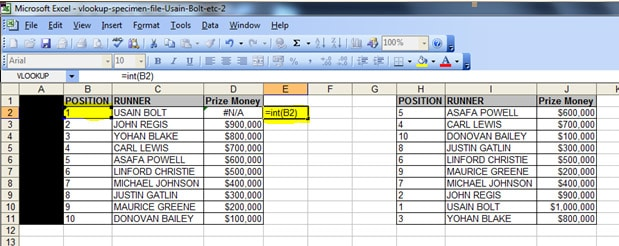#### 3) vlookup #N/A error (because wrong range of data is selected)

Here’s a problem from someone who visited this site (and the solution, of course!)

Rather than use the method described, they’d started by typing “=vlookup….” and had selected an incorrect range of cells to look at.

They said they had doubts about the vlookup function and didn’t know how to use it properly.

They were getting an n/a error and didn’t know why! So I got them to send me the file available on this site to see what they’d done wrong. There were 5 N/A errors in their spreadsheet but I’ve left only one instance of the error in the file, to make it easier for you to understand why it happened.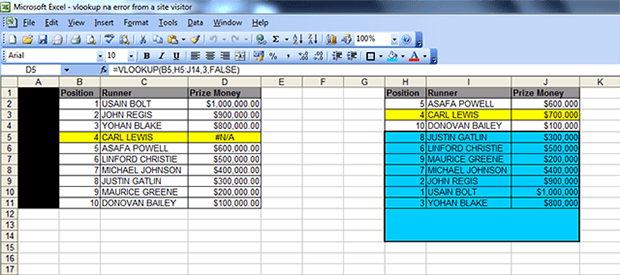The cell with the N/A error is cell D5 (see screenshot above). It has an “N/A” instead of the value “\$700,000”. You can clearly see that Carl Lewis is in position 4 in both tables (left and right); and if you look at the table on the right, the Prize Money assigned to him is \$700,000.

Here’s a link to the file with the error, in case you want to see it in closer detail – LINK TO FILE

The non-technical language explanation for this is that their vlookup formula was looking for a result for Carl Lewis in the BLUE AREA, when the result for Carl Lewis is in the YELLOW AREA ie ABOVE the BLUE AREA. (The vlookup was referencing the runner in position 4 but I have used the runner’s name as it’s quicker and easier to understand).

The technical explanation is this: the formula they created in cell D5 was this =VLOOKUP(B5,H5:J14,3,FALSE) where:

B5 is the runner in position 4 in the first table – which is ok (this is the lookup_value)

H5:J14 is the BLUE AREA  – which is NOT ok (this is the TABLE ARRAY)

3 is the column reference  – which is ok (this is the column_index_number)

FALSE – which is ok (this is called the  Range Lookup but it’s simply the last part of the formula to complete the argument and ensure an exact match is returned, and if not found, then an “N/A” is returned.

There are two solutions for this:

either use SOLUTION 1 which is =VLOOKUP(B5,H:J,3,FALSE)

OR use SOLUTION 2 which is: =VLOOKUP(B5,\$H\$2:\$J\$11,3,FALSE)

With SOLUTION 1, we are just changing the cell selection for the TABLE ARRAY from H5:J14 to H:J ie take out the numbers! Putting the wrong numbers in the TABLE ARRAY part of the formula will cause an “N/A”  error. In this case their formula started at cell H5 (which is position 8) but it is BELOW the area where the result they’re looking for (position 4) which is in the YELLOW AREA.

With SOLUTION 2, we are fixing the range of data from which the formula will search for a result. However, we cover all the “positions”, “runners” and “prize money” in the range. The visitor’s  range was missing the \$ signs and the cells H2 to J4.

So you can either highlight columns H to J in their entirety, as explained in the tutorials (see step 5 here: https://howtovlookupinexcel.com/) OR – highlight the range of cells of the data you’re referencing.

#### 4) vlookup #N/A error (because data is missing from table)

– this will happen either because the data you are looking up doesn’t exist (see table below where the numbers 1, 3 and 5 are not in the second table. You’ll notice that the ‘prize money for the runners in position 1, 3 and 5 in the first table is “N/A” instead of the actual value), so the formula returns an “N/A” instead of the result that you’re looking for.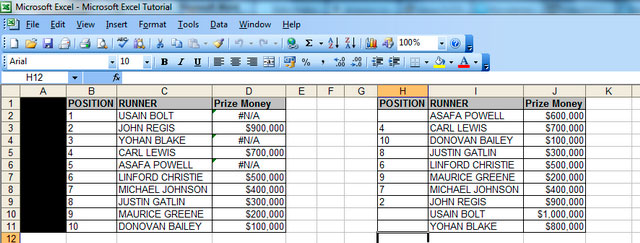– Or, alternatively, as above, the format of the data in your reference columns is ‘text’. You can fix this in one of two ways:

– i) the EASY way is to insert a column after your first reference column (so insert a column in between cells B and C, then type the following formula in cell C2:

=int(B2) then press ‘Enter’ (see screenshot below). After pressing ‘Enter’ drag down the formula so that all the cells in column B are made into ‘integers’ in column C. Now highlight the numbers in column C, copy them, then highlight the cells in column B and click ‘paste Special values’. Now delete column C, and your vlookup will work, like magic!!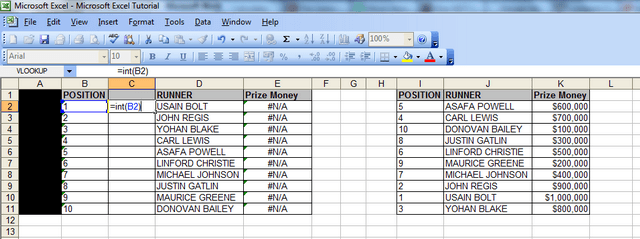– ii) the OTHER way is to change the format of each cell in col B to ‘general’, click ok, then press F2, then press Enter. (NB You must carry out those steps in exactly that order, otherwise they won’t work! Make sure after changing the format of the cell to ‘general’ and clicking ok, that you press F2 BEFORE pressing enter). However, with this method, you would have to do one cell at a time, which is time-consuming. I’m here to save you time!

#### 5) vlookup #N/A error because wrong ‘lookup value’ is used in the formula

In the formula in the screenshot below, you can see the formula

=VLOOKUP(M2,H:J,3,FALSE)

It has 4 different arguments which combined together create a vlookup:

=vlookup(lookup_value, table_array, col_index_num, [range_lookup])

However, the thing that’s wrong in this formula is the ‘lookup value’ – it is M2, when it should be B2. M2 is far away from both tables – see the cell highlighted in yellow. So simply changing M2 to B2 in this case will make the formula work ie the error is the first part of the formula, also known as the ‘lookup value’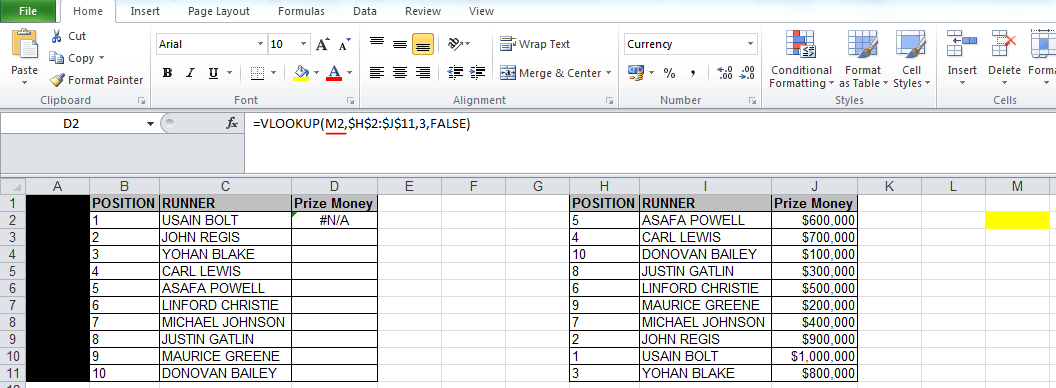#### 6) vlookup #REF! error

This will happen if the file, spreadsheet or table array that you were looking up data from has been deleted. An example of this is below, where I have deleted columns H to J and the vlookup described in the example above is returning the #REF! error.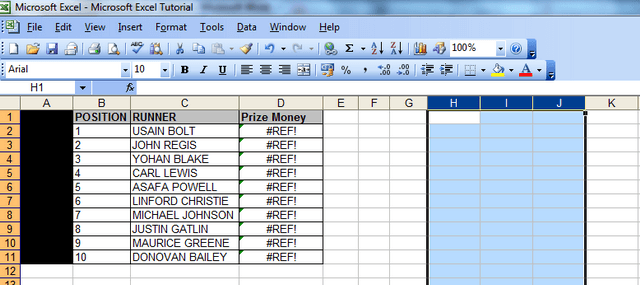#### 7) vlookup #REF error because table array is incorrect

In the screenshot below, the table array consists of 2 columns instead of 3 ie H to I instead of H to J.

The formula is trying to extract the “Prize Money” values in column J, so the formula needs to go up to column J to capture the information in that column!

Otherwise, the formula won’t work! Changing the letter I to J in this case will make the formula work.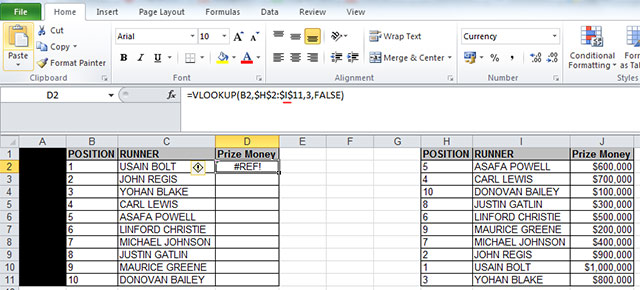#### 8)    vlookup not working for some cells/vlookup not working sometimes

–      if this happens, it is usually because your unique value is listed more than once in your reference table. And it would also have different values assigned to it. The screenshot below illustrates what I mean.

–      The number “4” appears twice in column H in the table on the right hand side, so the vlookup formula returns the first value assigned to the number “4” in that table, which happens to be \$10 (highlighted in red) in column J. If that row was deleted, then the vlookup would return the next value that it found assigned to the number “4” in that table, which would be \$700,000 (highlighted in yellow at the bottom). In this example, \$10 “Prize Money” is an unlikely amount for any athlete to get paid, as you can see all the other values are much higher, so the vlookup is returning the wrong result. To fix this, you would need to delete any duplicates that are irrelevant in your reference table.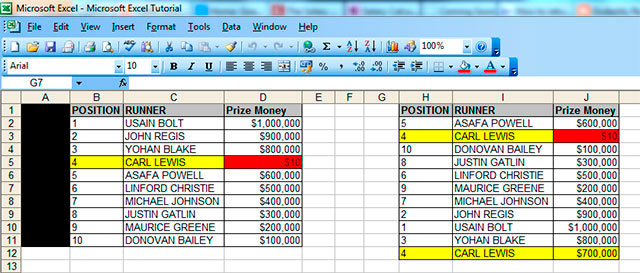–      to find out if a value is a duplicate in a spreadsheet that you’re working on, copy your unique value from your first table, in this case “4” in column B on the left hand side, go to your reference table or the other data that you are looking up (table on the right hand side above) and press ctrl+f then paste your unique value in the field that comes up shown below. Click on “Find Next” and Excel will move to each row where it can find the number “4” whenever you click “Find Next”. If it appears in the table more than once, then you’re likely to find that your vlookup is returning the wrong data.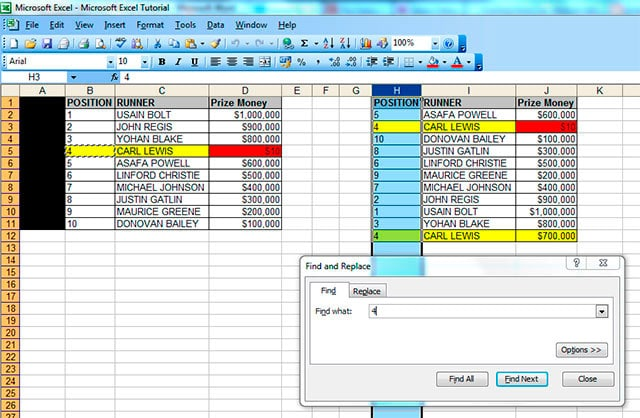#### 9) vlookup not working – just showing formula.

If your vlookup isn’t working, and is returning the formula like this (see cell D2)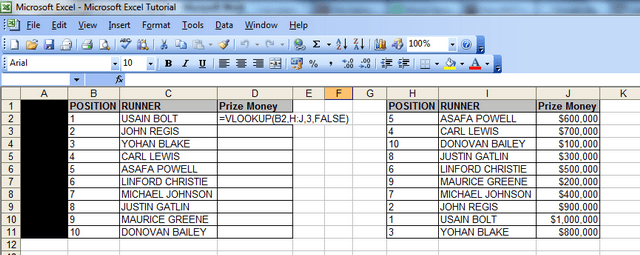then you need to

– left click into the cell where the formula isn’t working

– right click and choose ‘format cells’

– you will notice that the current format is ‘text’

– change this to ‘general’

– click ok

– then press F2

– then press ENTER. You need to ensure you press F2 before pressing enter, otherwise this won’t work.

– after you’ve done that, the problem will be fixed and you can drag the formula down to other cells in the column, if necessary.

#### 10) sum of sales data not working because some vlookups are returning N/As:

You may have experienced a problem at work where you are analysing data, and you have a spreadsheet with vlookups and a ‘sum function/total value formula’ at the bottom of each column with the vlookups. However, your sum function may be returning the word “N/A” instead of a total value or an actual figure.

You can fix this by changing your vlookups so that if they don’t find a value, they return a “0” instead of an “N/A”. The sum function will usually not work if there are “N/As” in the column concerned.
I have added in an extra row here (with Donald Trump as runner) to illustrate the above problem.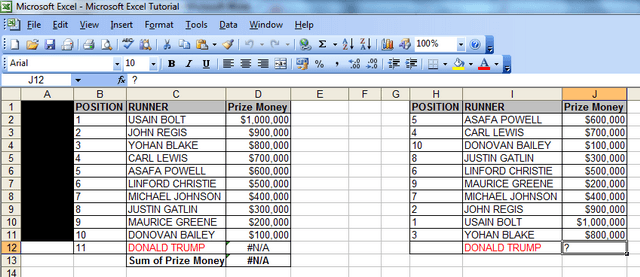To fix this, you need to add an IF statement to your vlookup. Let’s take the vlookup formula in cell D11 which currently looks like this: =VLOOKUP(B12,H:J,3,FALSE)

If you add the following text, the formula will return a “0” instead of an “NA”, and this will allow the sum function (=SUM(D2:D12)) in cell D13 to work. So just add these bits (I would suggest putting an apostrophe in front and writing this formula in a separate cell first):

i) ‘=IF(ISERROR

ii) copy and paste your original vlookup straight after the above ie (VLOOKUP(A2,I:M,5,FALSE)

iii) add the following ),0,

iv) then paste the original vlookup again (VLOOKUP(A2,I:M,5,FALSE) and add two brackets )) at the end to finish the formula (if you then take out the apostrophe and paste the formula into cell D11, the vlookup will return the value “0” and your sum function//totals formula will work.

v) the revised formula will look like this: =IF(ISERROR(VLOOKUP(A2,I:M,5,FALSE)),0,(VLOOKUP(A2,I:M,5,FALSE)))

The above formula basically says “if there is an error with the vlookup, return the number “0”, and if there isn’t an error, then return the value that the vlookup is looking for.

NEW UPDATE!!!

There is an alternative and SHORTER version of the above formula which can be used in Excel 2007 onwards – thanks to one of our visitors (Joe).

It uses the IFERROR function.

We’d simply just take the original function that we have ie (VLOOKUP(A2,I:M,5,FALSE) and add the word “=IFERROR” at the beginning and “,0)” at the end so it would look like this:

=IFERROR(VLOOKUP(A2,I:M,5,FALSE),0)

#### 11) #NAME error – because of incomplete ‘argument’ in the formula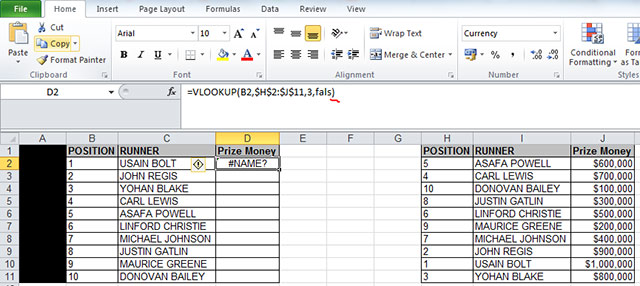In the example above, everything in the formula is fine except for the last word, which is “fals” instead of “false” with an “e” at the end.
So if you’re using words in formulas, it’s important to spell them correctly, otherwise you’ll get an #N/A error.

#### 12) vlookup not working when using a 2007 (or 2010) Excel file and you’re looking up data from an Excel 2003 file (or vice versa).

– in the Excel 2007 or 2010 file (s) [NOT 2003], click on ‘file’ then ‘save as’ then in the ‘save as type’ drop down menu at the bottom of the ‘save as’ pop up window, change file type from ‘Excel workbook (*.xlsx)’ – see screenshot below – to ‘Excel 97-2003 Workbook (.xls)’. You will then get the error message shown below in the second screenshot below, at which point, you click ‘yes’. Your vlookup between the files will now work!

#### 13) vlookup invalid error / vlookup invalid reference error

– If you’re working with large spreadsheets, ie those that have over 65,000 rows, you’re likely to come across the “vlookup invalid error” – the “invalid” message appears next to the table array field (see screenshot below). If you complete the vlookup anyway, you’ll then get another error message (see second screenshot below) which says “Invalid reference. This file version can only have formulas that reference cells within a worksheet size of 256 columns (column IW or higher) or 65,000 rows.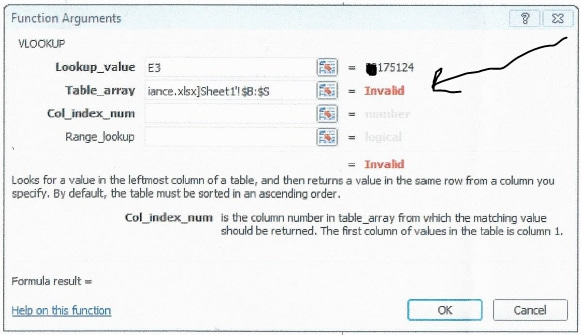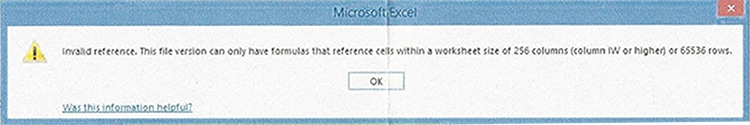The solution here is two-fold:

i)   Ensure that if your table array has more than 65,000 rows, you put anything between rows 65,0001 to 130,000 in a separate sheet, then put anything from rows 130,001 to 195,000 in a separate sheet, and so on, in increments of 65,000, because that’s the maximum number of rows the vlookup function will look it.

ii)   Ensure that rather than highlighting the columns (for your table array), you highlight the specific range of cells you’re looking up and “fix them” by pressing F4 – in the example on this site, the specific range for the table array would be cells H2 to J11 – you can see this by either downloading the spreadsheet – available at the top of the site; or simply looking at the screenshot in problem number 5 above.

Hope that’s solved all your problems! Have a nice day!